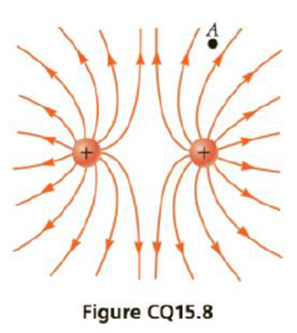Chapter 15, Problem 8CQ

Chapter
Section
Textbook Problem

Consider point A in Figure CQ15.8 located an arbitrary distance from two point charges in otherwise empty space. (a) Is it possible for an electric field to exist at point A in empty space? (b) Does charge exist at this point? (c) Does a force exist at this point?(a)

To determine
The electric field at point A.

Explanation

The electric field lines of a positive charge points outwards. The field of a positive charge points outwards. Both the positive charges will contribute to the net field at point A...

(b)

To determine
The charge at point A.

(c)

To determine
The force at point A.

Still sussing out bartleby?

Check out a sample textbook solution.

See a sample solution

The Solution to Your Study Problems

Bartleby provides explanations to thousands of textbook problems written by our experts, many with advanced degrees!

Get Started